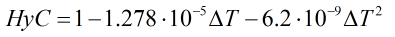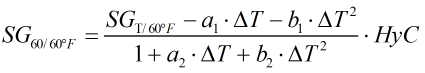# C199 – ASTM-IP Table 23:1952 – Specific Gravity Reduction to 60°F

## Description

This calculates the specific gravity 60/60°F from an observed specific gravity and corresponding temperature. Note that this calculation is only valid for the portion of Table 23 with specific gravity inputs of 0.600 and above.

Report on the Development, Construction, Calculation and Preparation of the ASTM-IP Petroleum Measurement Tables – ASTM D 1250; IP 200 (1960)

ASTM-IP Petroleum Measurement Tables – ASTM D 1250; IP 200 (1952)

Kelton calculation reference C199

FLOCALC calculation reference F085

## Options

### Precision

• Full
• Rounding

This option is used to choose whether to apply rounding to the same number of significant figures as the printed values in Petroleum Measurement Table 5 or output values with full precision.

### Hydrometer correction

• Apply

This option is chosen to apply the hydrometer correction described below.

## Calculation

### Hydrometer Correction

The hydrometer correction is calculated by:Where ΔT = Difference in temperature compared to base temperature in °F

### Specific Gravity 60/60°F

The specific gravity 60/60°F is calculated by an iterative process based around the relationship:Where ΔT = Difference in temperature compared to base temperature in °F SGT/60°F = specific gravity at observed temperature a1 = Constant from Table III a2 = Constant from Table III b1 = Constant from Table III b2 = Constant from Table III

Back